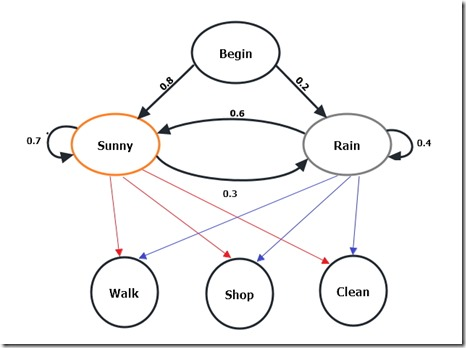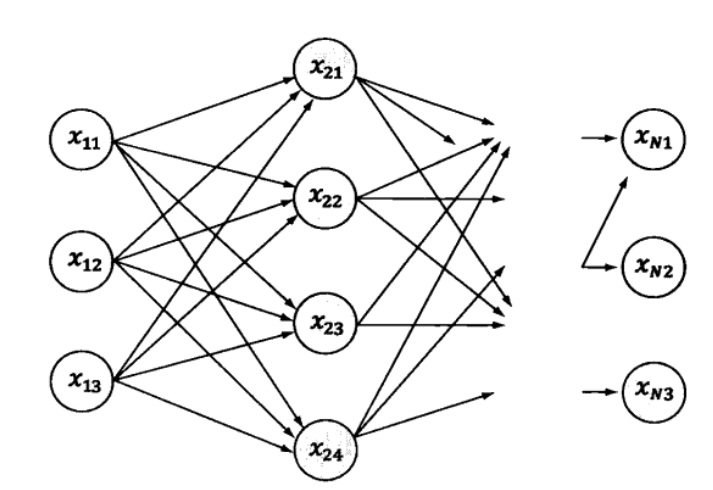﻿

# 隐马尔可夫模型（HMM）的原理

## 简介1. 知道整个模型后，你朋友告诉你他这三天的活动是：散步（Walk），购物（Shop），清洁屋子（Clean），那么根据模型，计算产生这些行为序列的概率是多少？
2. 知道整个模型后，朋友让你根据他的活动猜一猜他那边这三天天气怎么样
3. 朋友告诉你三天里他做了些什么，然后让你找出他活动规律的模型。

## 算法思路

### Forward Algorithm

t = 2 时，发生 “购物” 的概率，如果 t = 2 下雨，则$P(Walk_{t=1},Shop_{t=2},Rain_{t=2}) = [P(Walk_{t=1},Rain_{t=1}) \times P(Rain_{t=2}|Rain_{t=1}) \\ + P(Walk_{t=1},Sunny_{t=1})\times P(Rain_{t=2}|Sunny_{t=1})]\times P(Shop_{t=2}|Rain_{t=2}) \\ = [0.02*0.4+0.48*0.3]*0.4 = 0.0608$

$P(Walk_{t=1},Shop_{t=2},Sunny_{t=2}) = [0.02*0.6 +0.48*0.7]*0.3 = 0.1044$

t = 3 时的算法也可以依此类推，
$P(Walk_{t=1},Shop_{t=2},Clean_{t=3},Rain_{t=3}) = [0.0608*0.4+0.1044*0.3]*0.5 = 0.02782$
$P(Walk_{t=1},Shop_{t=2},Clean_{t=3},Sunny_{t=3}) = [0.0608*0.6+0.1044*0.7]*0.7 = 0.076692$

$P(Walk_{t=1},Shop_{t=2},Clean_{t=3}) = 0.02782 + 0.076692 = 0.104512$

### Backward Algorihm

$\beta_2(Rain) = a_{Rain \to Rain}b_{Rain}(O_3=Clean)\beta_3(Rain) \\+ a_{Rain \to Sunny}b_{Sunny}(O_3=Clean)\beta_3(Sunny)\\=0.4*0.5*1+0.6*0.1*1 = 0.26$

### Viterbi Algorithm（维比特算法）$x_1,x_2,...,x_N = \text{ArgMax}\left[P\left(x_1,x_2,...,x_N|y_1,y_2,...,y_N\right)\right(x \in X)]\\=\text{ArgMax}\left[\prod _{i=1}^N P\left(x_i|x_{i-1}\right)P\left(y_i|x_i\right)\right (x \in X)]$

1. 如果概率最大的路径P（或者说最优路径）经过某个点，如果上图中的$x_{22}$，那么这条路径上从起始点S 到 $x_{22}$的这一段子路径Q，一定是S到$x_{22}$的最短路径。否则用S到$x_{22}$的最短路径R来代替Q，便构成了一条比P更短的路径。

2. 从S到E的路径必定经过第i个时刻的某个状态，假定第i个时刻有k个状态，那么如果记录了从S到第i个状态的所以k个节点的最短路径，最终最短路径必经过其中的一条。这样，在任何时刻，只要考虑非常有限条最短路径即可。

3. 结合上述两点，假定当我们从状态 i 进入状态 i + 1 时，从 S 到状态 i 上各个节点的最短路径已经找到，并且记录在这些节点上，那么在计算从起点 S 到第 i + 1 状态的某个节点$x_{i+1}$的最短路径时，只要考虑从S到前一个状态 i 所有的 k 个节点的最短路径，以及从这 k 个节点到$x_{i+1}$，j 的距离即可。

#### 实现过程

1. 从点S出发，对于第一个状态$x_1$的各个节点，不妨假定有$n_1$个。计算出 S 到它们的距离$d(S，x_{1i})$,其中$x_{1i}$代表任意状态 1 的节点。因为只有一步，所以这些距离都是S到它们各自的最短距离。
2. 对于第二个状态$x_2$的所有节点，要计算出从S到它们的最短距离。我们知道，对于特定的节点$x_{2i}$，从S到它的路径可以经过状态1的$n_1$中任何一个节点$x_{1i}$，当然，对应的路径长度就是$d(S,x_{2i}) = d(S,x_{1j}) + d(x_{1j},x_{2j})$。由于 j 有$n_1$种可能性，我们要一一计算，然后找到最小值。即：$d(S,x_{2i}) = min_{i=1,n_1}d(S,x_{1j}) + d(x_{1j},x_{2j})$
3. 这样对于第二状态的每个节点，需要$n_1$次乘法计算。假定这个状态有$n_2$个节点，把S这些节点的距离都算一遍，就有$O(n_1 * n_2)$次计算。